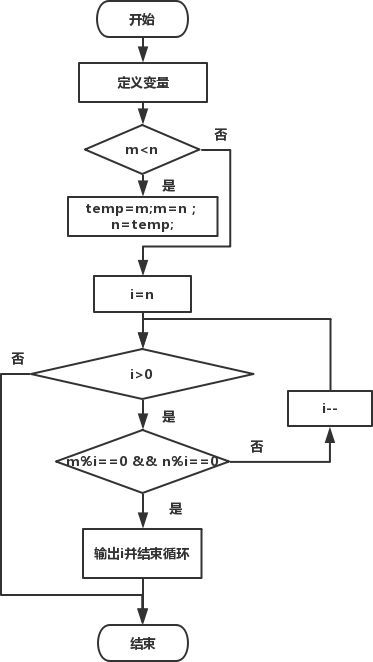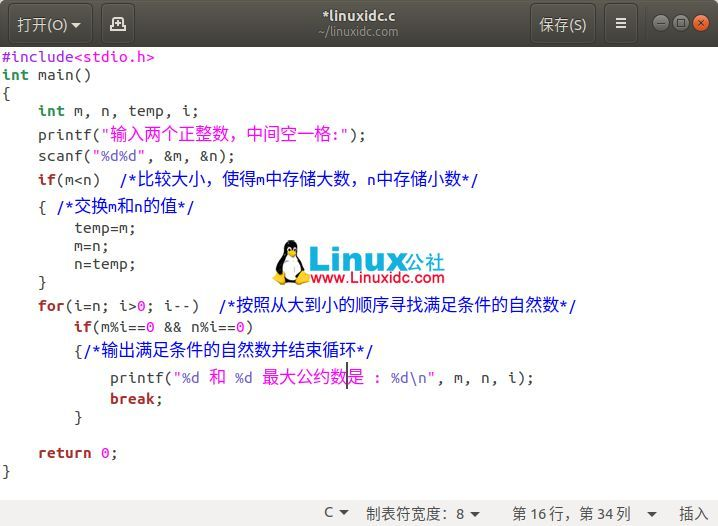•最大公约数
• C语言求最大公约数代码

万次阅读 多人点赞 2020-06-22 16:28:06
题目：随机输入两个数，最大公约数 在此展示三种常用解题思路 1.首先展示第一种思路 #include <stdio.h> int main() { int a,b,c; //先定义变量 printf("请输入：\n"); scanf("%d%d",&a,&b); ...

题目：随机输入两个数，求其最大公约数

在此展示三种常用解题思路
1.首先展示第一种思路

#include <stdio.h>
int main()
{
int a,b,c;             //先定义变量
printf("请输入：\n");
scanf("%d%d",&a,&b);   //输入两个整型数字
if(a<b)
{
c=a;
a=b;
b=c;               //保证a≥b
}
int i = 0;
for(i = b;i>0;i--)
{
if(a%i == 0 && b%i == 0)
{
printf("gcd(%d,%d) = %d\n",a,b,i);
break;
}
}
return 0;
}

2.辗转相除法
这里涉及到一个公式：GCD(a,b) = GCD(b,a%b)

#include <stdio.h>
int main()
{
int a,b,c;
printf("请输入：");
scanf("%d%d",&a,&b);
if(a<b)
{
c = a;
a = b;
b = c;                      //确保a≥b
}
while(b != 0)
{
c = b;
b = a%b;                    //辗转相除：相比第一种思路大大提高了代码的效率
a = c;
}
printf("最大公约数为：%d",a);
return 0;
}

还有一种运用递归的思想

#include <stdio.h>
void Gcd(int a,int b)
{
if(a > b)
{
if(a % b == 0)
{
return b;
}
else
{
return Gcd(b,a%b);
}
}
else
{
if(b % a == 0)
{
return a;
}
else
{
return Gcd(a,b%a);
}
}
}
int main()
{
int a, b;
int c = 0;
printf("请输入两个整数：");
scanf("%d%d",&a, &b);
c = Gcd(a, b);
printf("GCD（%d,%d）=%d\n",a,b,c);
}

3.更相减损术
定义：(如果需要对分数进行约分，那么)可以折半的话，就折半(也就是用2来约分)。如果不可以折半的话，那么就比较分母和分子的大小，用大数减去小数，互相减来减去，一直到减数与差相等为止，用这个相等的数字来约分。

#include <stdio.h>
main()
{
int a,b;
printf("请输入两个正整数：");
scanf("%d%d",&a,&b);
while(a%2==0)
{
a/=2;
}
while(b%2==0)
{
b/=2;
}               //让两个数都为奇数
while(a!=b)
{
if(a>b) a=a-b;
else b=b-a;              //更相减损术：当差值等于减数的值时即为最大公约数
}
printf("GCD=%d\n",a);
return 0;
}

本文结束，感谢阅览！

展开全文c语言
• 原标题：C语言求最大公约数代码及解析问题描述从键盘输入两个整数，任意两个正整数的最大公约数(GCD)。最大公因数，也称最大公约数、最大公因子，指两个或多个整数共有约数中最大的一个。a，b的最大公约数记为(a，...

原标题：C语言求最大公约数代码及解析

问题描述

从键盘输入两个整数，求任意两个正整数的最大公约数(GCD)。

最大公因数，也称最大公约数、最大公因子，指两个或多个整数共有约数中最大的一个。a，b的最大公约数记为(a，b)，同样的，a，b，c的最大公约数记为(a，b，c)，多个整数的最大公约数也有同样的记号。求最大公约数有多种方法，常见的有质因数分解法、短除法、辗转相除法、更相减损法。

问题分析

如果有一个自然数a能被自然数b整除，则称a为b的倍数，b为a的约数。几个自然数公有的约数，叫做这几个自然数的公约数。公约数中最大的一个公约数，称为这几个自然数的最大公约数。

根据约数的定义可知，某个数的所有约数必不大于这个数本身，几个自然数的最大公约数必不大于其中任何一个数。要求任意两个正整数的最大公约数即求出一个不大于其中两者中的任何一个，但又能同时整除两个整数的最大自然数。

算法设计

思路有两种：第一种，采用穷举法按从小到大(初值为1，最大值为两个整数当中较小的数)的顺序将所有满足条件的公约数列出，输出其中最大的一个；第二种，按照从大(两个整数中较小的数)到小(到最小的整数1)的顺序求出第一个能同时整除两个整数的自然数，即为所求。

下面对第二种思路进行详细说明。

两个数的最大公约数有可能是其中的小数，所以在按从大到小顺序找寻最大公约数时，循环变量i的初值从小数n开始依次递减，去寻找第一个能同时整除两整数的自然数，并将其输出。需要注意的是，虽然判定条件是i>0，但在找到第一个满足条件的i值后，循环没必要继续下去，如，25和15，最大公约数是5，对于后面的4、3、2、1没必要再去执行，但此时判定条件仍然成立，要结束循环只能借助break语句。

程序流程图：下面是完整的代码：

#include

int main()

{

int m, n, temp, i;

printf("输入两个正整数，中间空一格:");

scanf("%d%d", &m, &n);

if(m

{ /*交换m和n的值*/

temp=m;

m=n;

n=temp;

}

for(i=n; i>0; i--) /*按照从大到小的顺序寻找满足条件的自然数*/

if(m%i==0 && n%i==0)

{/*输出满足条件的自然数并结束循环*/

printf("%d 和 %d 最大公约数是 : %dn", m, n, i);

break;

}

return 0;

}运行结果：

输入两个正整数，中间空一格:100 150

150 和 100 最大公约数是 : 50

本文永久更新链接地址：https://www.linuxidc.com/Linux/2018-11/155461.htm返回搜狐，查看更多

责任编辑：

展开全文最大公约数c语言
• 求最大公因数C语言

千次阅读 2019-11-08 09:13:12
辗转相除法： #include<stdio.h>//辗转相除法 int fcg(int m,int n) { int r=m%n; while(r!=0) { m=n; n=r; r=m%n; } printf("%d",n); } int main() { int a,b; scanf("%d%d",&a,&......

辗转相除法：

#include<stdio.h>//辗转相除法
int fcg(int m,int n)
{
int r=m%n;
while(r!=0)
{
m=n;
n=r;
r=m%n;

}
printf("%d",n);

}
int main()
{
int a,b;
scanf("%d%d",&a,&b);
if(a<b)
{
int t;
t=a;
a=b;
b=t;
}
fcg(a,b);

}

相减法：

#include<stdio.h>//相减法
int main()
{
int a,b;
scanf("%d%d",&a,&b);
while(a!=b)
{
if(a>b)
{
a=a-b;

}
else
{
b=b-a;
}
}
printf("%d",a);

}

穷举法：

#include<stdio.h>//穷举法
int main()
{
int a,b;
scanf("%d%d",&a,&b);
if(a<b)
{
int t;
t=a;
a=b;
b=t;
}
int t;
for(t=b;t>=1;t–)
{
if(b%t0&&a%t0)
{
printf("%d",t);
break;
}
}
}

展开全文C语言
• include <stdio.h> int main() { int i,j; int min; if(i>j) { min=j; } else i=j; j=min; scanf("%d%d",&i,&j); for(minj;min>0;min–) { if(i%min0&&j%min==0) ...}

include <stdio.h>

int main()
{
int i,j;
int min;
if(i>j)
{
min=j;
}
else
i=j;
j=min;
scanf("%d%d",&i,&j);
for(min=j;min>0;min–)
{
if(i%min0&&j%min0)
{
printf("%d\n",min);
break;
}
}

return 0;
}

展开全文• 代码如下： int gcd( int x, int y ){ int divisor = x % y; while(divisor != 0){ //辗转相除法 x = y; y = divisor;... } //循环结束之后， y为最大公约数 divisor = y; return divisor; }
• 设计MaxCommMultiple()，计算两个正整数的最大公约数最大公约数
• 求最大公约数（C语言实现)

万次阅读 多人点赞 2020-12-17 20:35:48
求最大公约数有多种方法，接下来我对辗转相除法、更相减损法分别做介绍。 辗转相除法： gcd(a,b) = gcd(b,a mod b)。 假如，需要求 1997 和 615 两个正整数的最大公约数,用辗转相除法，是这样进行的： 1997 / 615 = ...算法
• b），它们的最大公约数等于a除以b的余数c和b之间的最大公约数代码实现： #include <stdio.h> int gcd(int a, int b) { if (a % b == 0) { return b; } else { return gcd(b, a % b); } } int ...c语言 开发语言 后端
• //注意最大公约数(m)与最小公倍数(n)的关系 #include<stdio.h> int main(){ int m,n,t,c,s; scanf("%d %d",&m,&n); if(m<n){ t=m; m=n; n=t; } s=m*n; c=m%n; while(c!=0){ m=n; n=c; c=m%n; } ...c语言
• 两个数的最大公约数即为可以被两个数整除的最大值，所以会有两种情况。一种是不等于输入的两个值。一种是等于两个数中最小的那个。所以代码用到if语句进行第一次判断。第二次判断则写在for语句中进行递减数循环条件...
• 穷举法求最大公约数C语言

千次阅读 2020-11-17 06:32:07
穷举法求最大公约数 问题描述 尝试基于以下逻辑编程计算最大公约数：由于a和b的最大公约数不可能比a和b中的较小者还大，否则一定不能整除它，因此，先找到a和b中的较小者t，然后从t开始逐次减1尝试每种可能，即检验...c语言
• #include <stdio.h> int main() { int a = 0; int b = 0; int tep = 0; printf("请输入两个数"); scanf("%d %d", &a, &b); while (tep = a%b) { a = b; b = tep; } ......c语言 c++
• 学习写了很多种最大公因数代码，还是看MOOC翁恺老师的代码最简洁。 辗转相除法： 又称欧几里得算法，用于计算两个非负整数的最大公约数。以除数和余数反复做除法运算，当余数为0时，取当前式子除数为最大公...c语言
• C语言求最大公约数(递归)

千次阅读 2020-10-26 19:44:55
辗转相除法 int gcd(int a,int b) { if(a%b==0) { printf("%d",b); return 0; } gcd(b,a%b); } #include <stdio.h> int main() { int a=24,b=10,i; if(a<b) //用辗转相除法要保证a>...4(a%b)%2(b%(a
• a=nc,b=mc，那么a-b=(n-m)c，从等式可以看出，a,b两个数的最大公约数，就相当于b,(a-b)两个数的最大公约数。如此递推下去，总. #include int MaxCommonFactor(int a,int b) {int c; if(areturn -1; else if(ac=a...
• 欧几里德算法又称辗转相除法,欧几里德算法是用来两个正整数最大公约数的算法。古希腊数学家欧几里德在其著作《The Elements》中最早描述了这种算法,所以被命名为欧几里德算法。扩展欧几里德算法可用于RSA加密等...公约数 C语言 C语言编程 C语言学习 C语言试题
• 用C语言递归求最大公约数

万次阅读 2018-05-23 22:03:40
但是之前用辗转相除法求最大公约数是不是不够方便？用递归实现代码简单；而且思路也简单：int f(int m,int n){ if(m%n == 0)return n; else return f(n,m%n); }这是关键代码，f是函数，在函数内又调用自身；...C语言 递归 求最大公约数
• /*求最大公约数，最小公倍数的 */#include int maxdivisor(int ,int );/*求最大公约数函数*/int minmultiple(int ,int ,int );/*最小公约数函数*/int dazaiqian(int *,int *);/*交换两个数，使大的在前的那个变量*...
• c语言递归求最大公约数

千次阅读 2021-01-19 12:45:24
两个正整数m和n的最大公约数。 输入样例1： 6 8 输出样例1： 2 //递归求最大公约数 #include<stdio.h> int f(int a,int b) { //比大小,确定被除数和除数 //a为被除数，b为除数 if(b>a) { int ...
• C语言四种方法求最大公约数

万次阅读 多人点赞 2019-03-09 13:26:03
1.辗转相除法（欧几里德法） C语言中用于计算两个正整数a,b的最大公约数，采用函数嵌套调用形式进行两个数的最大公约数。其算法过程为： 前提：设两数为a,b设其中a做被除数,b做除数,temp为余数 Steps:大数放a中...
• 下面上代码： #include<stdio.h> #define max(a,b) ((a)>(b)?(a):(b)) #define min(a,b) ((a)<(b)?(a):(b)) int getGCD(int a,int b){ return a == b ? a : getGCD(min(a,b),max(a,b)-min(a,b)); } ...c语言 递归算法
• 1062: 最大公约数 时间限制: 1 Sec 内存限制: 128 MB 提交: 32135 解决: 19164 [状态] [讨论版] [提交] [命题人:admin] 题目描述 输入两个不大于10的9次方的正整数，输出其最大公约数。 输入 输入两个正整数m和n，...c语言 算法
• int gcd(int a, int b){ // 最大公约数 return !b ? a : gcd(b, a%b); } int lcm(int a, int b){ //最小公倍数 return a/gcd(a,b)*b; }
• C语言递归求最大公约数 #include<stdio.h> int gcd(int a,int b); int main() { int a,b; printf(“输入两整数：”); scanf("%d,%d",&a,&b); printf(“最大公约数是%d”,gcd(a,b)); return 0; } int ...
• 这是一个C语言 while 循环示例：正整数 m 和 n 的最大公约数。问题分析输入：两个正整数。输出：一个正整数(最大公约数)。最大公约数(gcd)是指几个数共有的因数之中最大的一个数，比如 8 和 12 的最大公约数是 4，...
• 课程的随堂作业，C语言的，用dev就能运行，萌新代码，勿喷，仅仅帮助不想写作业的朋友方便一下，反正老师也不会仔细检查的
• C语言求最大公约数GCD的算法C语言求最大公约数GCD的算法完整源码(定义，实现，main函数测试） C语言求最大公约数GCD的算法完整源码(定义，实现，main函数测试） #include <iostream> int gcd1( int a, int b...
• 第一种：用较小数的最大约数于较大数作模元算#include/*两个数的最大公约数*/intmain(){inta,b,max,min,i,result;scanf("%d,%d",&a,&b);printf("您输入的的值分别为%d,%d\n",a,b);if(a>b){max=a;min=...c语言最大公约数简单...

求最大公约数c语言代码c语言 订阅